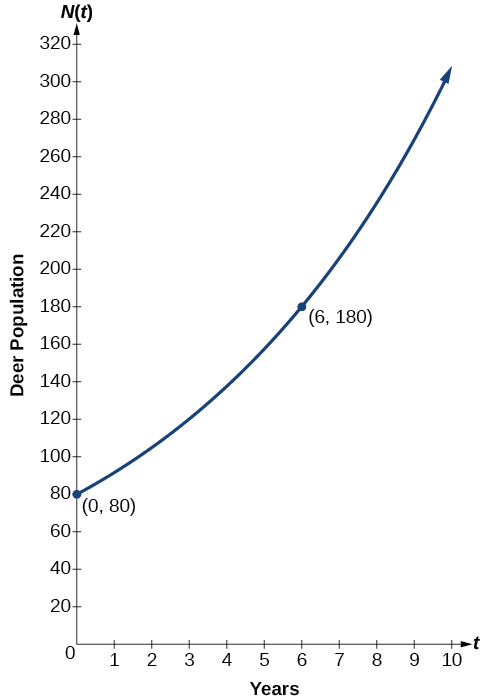# 6.1 Exponential functions  (Page 4/16)

 Page 4 / 16

## Evaluating a real-world exponential model

At the beginning of this section, we learned that the population of India was about $\text{\hspace{0.17em}}1.25\text{\hspace{0.17em}}$ billion in the year 2013, with an annual growth rate of about $\text{\hspace{0.17em}}1.2%.\text{\hspace{0.17em}}$ This situation is represented by the growth function $\text{\hspace{0.17em}}P\left(t\right)=1.25{\left(1.012\right)}^{t},$ where $\text{\hspace{0.17em}}t\text{\hspace{0.17em}}$ is the number of years since $\text{\hspace{0.17em}}2013.\text{\hspace{0.17em}}$ To the nearest thousandth, what will the population of India be in $\text{\hspace{0.17em}}\text{2031?}$

To estimate the population in 2031, we evaluate the models for $\text{\hspace{0.17em}}t=18,$ because 2031 is $\text{\hspace{0.17em}}18$ years after 2013. Rounding to the nearest thousandth,

$P\left(18\right)=1.25{\left(1.012\right)}^{18}\approx 1.549$

There will be about 1.549 billion people in India in the year 2031.

The population of China was about 1.39 billion in the year 2013, with an annual growth rate of about $\text{\hspace{0.17em}}0.6%.\text{\hspace{0.17em}}$ This situation is represented by the growth function $\text{\hspace{0.17em}}P\left(t\right)=1.39{\left(1.006\right)}^{t},$ where $\text{\hspace{0.17em}}t\text{\hspace{0.17em}}$ is the number of years since $\text{\hspace{0.17em}}2013.$ To the nearest thousandth, what will the population of China be for the year 2031? How does this compare to the population prediction we made for India in [link] ?

About $\text{\hspace{0.17em}}1.548\text{\hspace{0.17em}}$ billion people; by the year 2031, India’s population will exceed China’s by about 0.001 billion, or 1 million people.

## Finding equations of exponential functions

In the previous examples, we were given an exponential function, which we then evaluated for a given input. Sometimes we are given information about an exponential function without knowing the function explicitly. We must use the information to first write the form of the function, then determine the constants $\text{\hspace{0.17em}}a\text{\hspace{0.17em}}$ and $\text{\hspace{0.17em}}b,$ and evaluate the function.

Given two data points, write an exponential model.

1. If one of the data points has the form $\text{\hspace{0.17em}}\left(0,a\right),$ then $\text{\hspace{0.17em}}a\text{\hspace{0.17em}}$ is the initial value. Using $\text{\hspace{0.17em}}a,$ substitute the second point into the equation $\text{\hspace{0.17em}}f\left(x\right)=a{\left(b\right)}^{x},$ and solve for $\text{\hspace{0.17em}}b.$
2. If neither of the data points have the form $\text{\hspace{0.17em}}\left(0,a\right),$ substitute both points into two equations with the form $\text{\hspace{0.17em}}f\left(x\right)=a{\left(b\right)}^{x}.\text{\hspace{0.17em}}$ Solve the resulting system of two equations in two unknowns to find $\text{\hspace{0.17em}}a\text{\hspace{0.17em}}$ and $\text{\hspace{0.17em}}b.$
3. Using the $\text{\hspace{0.17em}}a\text{\hspace{0.17em}}$ and $\text{\hspace{0.17em}}b\text{\hspace{0.17em}}$ found in the steps above, write the exponential function in the form $\text{\hspace{0.17em}}f\left(x\right)=a{\left(b\right)}^{x}.$

## Writing an exponential model when the initial value is known

In 2006, 80 deer were introduced into a wildlife refuge. By 2012, the population had grown to 180 deer. The population was growing exponentially. Write an algebraic function $\text{\hspace{0.17em}}N\left(t\right)\text{\hspace{0.17em}}$ representing the population $\text{\hspace{0.17em}}\left(N\right)\text{\hspace{0.17em}}$ of deer over time $\text{\hspace{0.17em}}t.$

We let our independent variable $\text{\hspace{0.17em}}t\text{\hspace{0.17em}}$ be the number of years after 2006. Thus, the information given in the problem can be written as input-output pairs: (0, 80) and (6, 180). Notice that by choosing our input variable to be measured as years after 2006, we have given ourselves the initial value for the function, $\text{\hspace{0.17em}}a=80.\text{\hspace{0.17em}}$ We can now substitute the second point into the equation $\text{\hspace{0.17em}}N\left(t\right)=80{b}^{t}\text{\hspace{0.17em}}$ to find $\text{\hspace{0.17em}}b:$

NOTE: Unless otherwise stated, do not round any intermediate calculations. Then round the final answer to four places for the remainder of this section.

The exponential model for the population of deer is $\text{\hspace{0.17em}}N\left(t\right)=80{\left(1.1447\right)}^{t}.\text{\hspace{0.17em}}$ (Note that this exponential function models short-term growth. As the inputs gets large, the output will get increasingly larger, so much so that the model may not be useful in the long term.)

We can graph our model to observe the population growth of deer in the refuge over time. Notice that the graph in [link] passes through the initial points given in the problem, and We can also see that the domain for the function is $\text{\hspace{0.17em}}\left[0,\infty \right),$ and the range for the function is $\text{\hspace{0.17em}}\left[80,\infty \right).$Graph showing the population of deer over time,   N ( t ) = 80 ( 1.1447 ) t , t   years after 2006

#### Questions & Answers

write down the polynomial function with root 1/3,2,-3 with solution
if A and B are subspaces of V prove that (A+B)/B=A/(A-B)
write down the value of each of the following in surd form a)cos(-65°) b)sin(-180°)c)tan(225°)d)tan(135°)
Prove that (sinA/1-cosA - 1-cosA/sinA) (cosA/1-sinA - 1-sinA/cosA) = 4
what is the answer to dividing negative index
In a triangle ABC prove that. (b+c)cosA+(c+a)cosB+(a+b)cisC=a+b+c.
give me the waec 2019 questions
the polar co-ordinate of the point (-1, -1)
prove the identites sin x ( 1+ tan x )+ cos x ( 1+ cot x )= sec x + cosec x
tanh`(x-iy) =A+iB, find A and B
B=Ai-itan(hx-hiy)
Rukmini
what is the addition of 101011 with 101010
If those numbers are binary, it's 1010101. If they are base 10, it's 202021.
Jack
extra power 4 minus 5 x cube + 7 x square minus 5 x + 1 equal to zero
the gradient function of a curve is 2x+4 and the curve passes through point (1,4) find the equation of the curve
1+cos²A/cos²A=2cosec²A-1
test for convergence the series 1+x/2+2!/9x3ByByBy DanielrosenbergerBy